# 前言

you.come() ? rain.stop() : I.wait()

# L1-1  人与神 (5 分)

### 输入样例：

``无``

### 输出样例：

``To iterate is human, to recurse divine.``

``````#include<iostream>;
#include<bits/stdc++.h>
#include<algorithm>
using namespace std;
int main(){
cout<<"To iterate is human, to recurse divine.";
return 0;
}``````

# L1-2 两小时学完C语言 (5 分)

### 输入样例：

``100000 1000 72``

### 输出样例：

``28000``

``````#include<iostream>;
#include<bits/stdc++.h>
#include<algorithm>
using namespace std;
int main() {
int n,k,m;
cin>>n>>k>>m;
cout<<n-k*m;
return 0;
}``````

# L1-3 强迫症 (10 分)

### 输入样例 1：

``9808``

### 输出样例 1：

``1998-08``

### 输入样例 2：

``0510``

### 输出样例 2：

``2005-10``

### 输入样例 3：

``196711``

### 输出样例 3：

``1967-11``

``````#include<iostream>
#include<bits/stdc++.h>
#include<algorithm>
using namespace std;

string s;

int main() {
cin>>s;
int year;
int len=s.length() ;
year=(s-48)*10+(s-48);
if(len==4) {
if(year<22) cout<<"20";
else		cout<<"19";
cout<<s<<s<<'-'<<s<<s;
} else {
for(int i=0; i<4; i++)	cout<<s[i];
cout<<'-';
for(int i=4; i<6; i++)	cout<<s[i];
}
return 0;
}``````

# L1-4 降价提醒机器人 (10 分)

### 输入样例：

``````4 99
98.0
97.0
100.2
98.9``````

### 输出样例：

``````On Sale! 98.0
On Sale! 97.0
On Sale! 98.9``````

``````#include<iostream>
#include<bits/stdc++.h>
#include<algorithm>
using namespace std;

int main() {
int n;
double m,num;
cin>>n>>m;
while(n--) {
cin>>num;
if(num<m) {
cout<<"On Sale! ";
printf("%.1lf\n",num);
}
}
return 0;
}``````

# L1-5 大笨钟的心情 (15 分)### 输入样例：

``````80 75 60 50 20 20 20 20 55 62 66 51 42 33 47 58 67 52 41 20 35 49 50 63
17
7
3
15
-1``````

### 输出样例：

``````52 Yes
20 No
50 No
58 Yes``````

``````#include<iostream>
#include<bits/stdc++.h>
#include<algorithm>
using namespace std;

int lis;
int num;

int main() {
for(int i=0; i<24; i++)	cin>>lis[i];
while(1) {
cin>>num;
if(num>=0&&num<=23) {
cout<<lis[num]<<' ';
lis[num]>50?cout<<"Yes":cout<<"No";
cout<<endl;
} else	break;
}
return 0;
}``````

# L1-6 吉老师的回归 (15 分)

### 输入样例 1：

``````5 1
L1-1 is a qiandao problem.
L1-2 is so...easy.
L1-3 is Easy.
L1-4 is qianDao.
Wow, such L1-5, so easy.``````

### 输出样例 1：

``L1-4 is qianDao.``

### 输入样例 2：

``````5 4
L1-1 is a-qiandao problem.
L1-2 is so easy.
L1-3 is Easy.
L1-4 is qianDao.
Wow, such L1-5, so!!easy.``````

### 输出样例 2：

``Wo AK le``

STL真好用，不用手写KMP算法。

PS：不过python更BUG，直接判断（in） 。

``````#include<iostream>
#include<bits/stdc++.h>
#include<algorithm>
#include<vector>
#include<cstring>
using namespace std;
typedef long long ll;

string qd="qiandao";
string ez="easy";

string::iterator it1,it2;

int n,m,cnt;

struct {
string name;
} lis;

int main() {
cin>>n>>m;
getchar();
for(int i=0; i<n; i++) {
getline(cin,lis[i].name);
}
for(int i=0; i<n; i++) {
it1=search(lis[i].name.begin() ,lis[i].name.end(),qd.begin() ,qd.end() );
it2=search(lis[i].name.begin() ,lis[i].name.end(),ez.begin() ,ez.end() );
if(it1==lis[i].name.end() &&it2==lis[i].name.end() ) {
cnt++;
}
if(cnt==m)	{
i++;
while(i<n) {
it1=search(lis[i].name.begin() ,lis[i].name.end(),qd.begin() ,qd.end() );
it2=search(lis[i].name.begin() ,lis[i].name.end(),ez.begin() ,ez.end() );
if(it1==lis[i].name.end() &&it2==lis[i].name.end() ) {
cout<<lis[i].name;
return 0;
}
i++;
}
}
}
cout<<"Wo AK le";
return 0;
}``````

# L1-7 天梯赛的善良 (20 分)

### 输入样例：

``````10
86 75 233 888 666 75 886 888 75 666``````

### 输出样例：

``````75 3
888 2``````

STL函数。

``````#include<iostream>
#include<bits/stdc++.h>
#include<algorithm>
#include<vector>
using namespace std;

typedef long long ll;

ll n,m;
vector<ll>s;
ll lis;
ll ans;
int  main(){
ll n;
cin>>n;
for(ll i=0;i<n;i++)	cin>>lis[i];
ans=*min_element(lis,lis+n);
cout<<ans<<' '<<count(lis,lis+n,ans)<<endl;
ans=*max_element(lis,lis+n);
cout<<ans<<' '<<count(lis,lis+n,ans);
return 0;
}``````

# L1-8 乘法口诀数列 (20 分)

### 输入样例：

``2 3 10``

### 输出样例：

``2 3 6 1 8 6 8 4 8 4``

### 样例解释：

STL模拟，动态数组大小有n就行了。

``````#include<iostream>
#include<bits/stdc++.h>
#include<algorithm>
#include<vector>
using namespace std;
typedef long long ll;

vector<int>s;

int main(){
int a1,a2;
unsigned int n;
cin>>a1>>a2>>n;
s.push_back(a1);
s.push_back(a2);
int len=s.size() ;
int it=0;
while(s.size()<=n){
int c=s[it]*s[it+1];
char t;
sprintf(t,"%d",c);
for(int i=0;t[i];i++)	s.push_back(t[i]-48);
len=s.size() ;
it++;
}
for(int i=0;i<n;i++){
cout<<s[i];
if(i!=n-1)	cout<<' ';
}
return 0;
}``````

# L2-1 包装机 (25 分)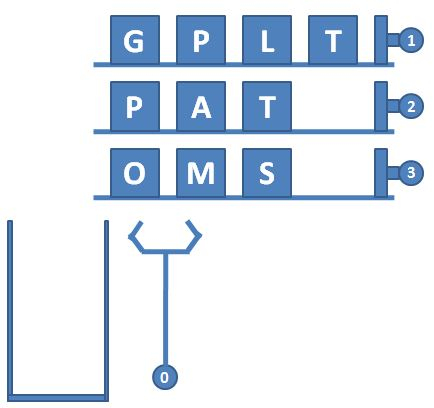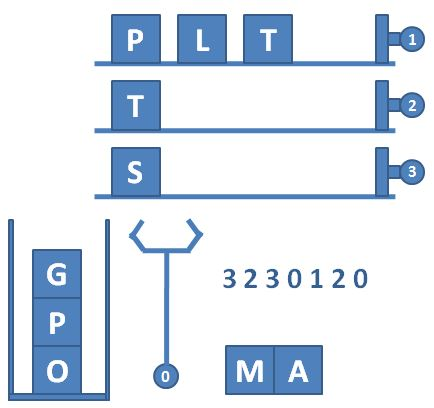### 输入样例：

``````3 4 4
GPLT
PATA
OMSA
3 2 3 0 1 2 0 2 2 0 -1``````

### 输出样例：

``MATA``

``````#include<iostream>
#include<bits/stdc++.h>
#include<algorithm>
#include<vector>
#include<stack>
using namespace std;
typedef long long ll;

ll n,m;
unsigned long long maxn;
ll flag;
char c;

struct {
int pos=0;
string gui;
} lis;

stack<char> s;
vector<char> ans;

int main() {
cin>>n>>m>>maxn;
for(ll i=1; i<=n; i++) {
lis[i].pos=0;
cin>>lis[i].gui;
}

cin>>flag;
while(flag!=-1) {
if(flag==0) {
if(s.size() !=0) {
ans.push_back(s.top() ) ;
s.pop() ;
}
} else {
if(lis[flag].pos<m) {
c=lis[flag].gui[lis[flag].pos++];
if(s.size() >=maxn) {
ans.push_back(s.top() ) ;
s.pop() ;
s.push(c) ;
} else {
s.push(c) ;
}
}
}
cin>>flag;
}

for(auto &it:ans)	cout<<it;

return 0;
}``````

# L2-2 病毒溯源 (25 分)### 输入格式：

``k 变异株1 …… 变异株k``

### 输入样例：

``````10
3 6 4 8
0
0
0
2 5 9
0
1 7
1 2
0
2 3 1``````

### 输出样例：

``````4
0 4 9 1``````

# L2-3 清点代码库 (25 分)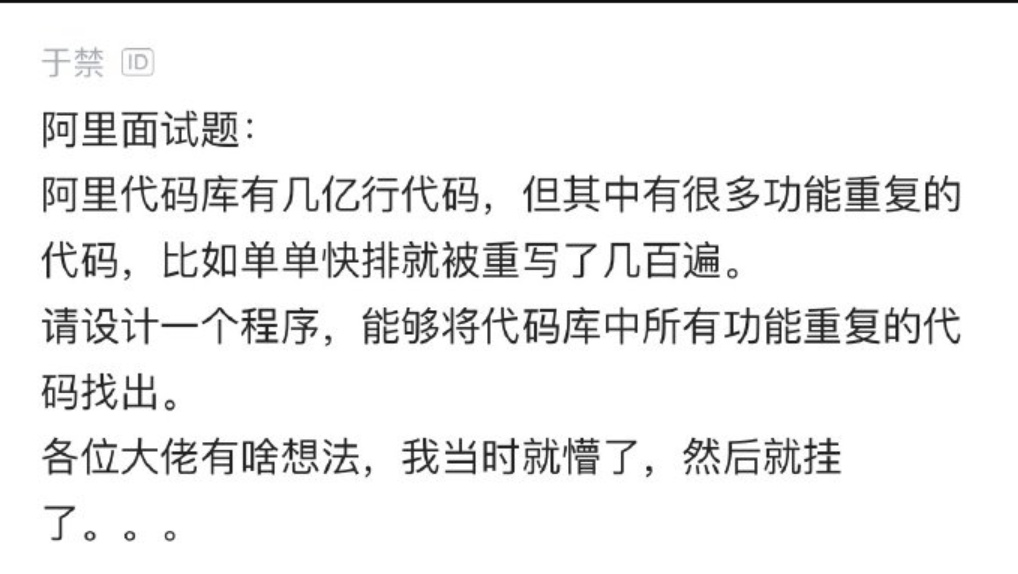### 输入样例：

``````7 3
35 28 74
-1 -1 22
28 74 35
-1 -1 22
11 66 0
35 28 74
35 28 74``````

### 输出样例：

``````4
3 35 28 74
2 -1 -1 22
1 11 66 0
1 28 74 35``````

``````#include<iostream>
#include<bits/stdc++.h>
#include<algorithm>
#include<vector>
#include<stack>
#include<map>
#include<string>
using namespace std;
typedef long long ll;

ll x=0,f=1;
char c=getchar();
while(c<'0'||c>'9') {
if(c=='-')	f=-1;
c=getchar();
}
while(isdigit(c))	x=x*10+(c-48),c=getchar();
return x*f;
}

typedef struct {
vector<ll>sc;
ll cnt;
} TYPELIS;

vector<ll>t;
ll num;
map<vector<ll>,int>mp;

/*
bool cmp(TYPELIS a,TYPELIS b) {
if(a.cnt==b.cnt)	return a.sc[a.sc.size()-1]<b.sc[b.sc.size()-1];
return a.cnt>b.cnt;
}
*/

bool cmp(TYPELIS a,TYPELIS b) {
if(a.cnt==b.cnt) {
for(unsigned  int i=0; i<a.sc.size() ; i++) {
if(a.sc[i]==b.sc[i])	continue;
return a.sc[i]<b.sc[i];
}
}
return a.cnt>b.cnt;
}

ll n,m;

int main() {

TYPELIS lis[n+10];

for(ll i=0; i<n; i++) {
for(ll j=0; j<m; j++) {
t[i].push_back(num) ;
}
mp[t[i]]++;
}

ll len=0;
for(auto &it:mp) {
lis[len].sc=it.first;
lis[len].cnt=it.second;
len++;
}

cout<<len<<endl;

sort(lis,lis+len,cmp);

for(ll i=0; i<len; i++) {
cout<<lis[i].cnt<<' ';
int len=lis[i].sc.size();
for(ll j=0; j<len; j++) {
cout<<lis[i].sc[j];
if(j!=len-1)	cout<<' ';
}
cout<<endl;
}

return 0;
}``````

# L2-4 哲哲打游戏 (25 分)

### 输入格式：

• 0 表示哲哲做出了某个操作或选择，后面紧接着一个数字 j，表示哲哲在当前剧情点做出了第 j 个选择。我们保证哲哲的选择永远是合法的。
• 1 表示哲哲进行了一次存档，后面紧接着是一个数字 j，表示存档放在了第 j 个档位上。
• 2 表示哲哲进行了一次读取存档的操作，后面紧接着是一个数字 j，表示读取了放在第 j 个位置的存档。

### 输入样例：

``````10 11
3 2 3 4
1 6
3 4 7 5
1 3
1 9
2 3 5
3 1 8 5
1 9
2 8 10
0
1 1
0 3
0 1
1 2
0 2
0 2
2 2
0 3
0 1
1 1
0 2``````

### 输出样例：

``````1
3
9
10``````

### 样例解释：

1 -> 4 -> 3 -> 7 -> 8 -> 3 -> 5 -> 9 -> 10。

``````#include<iostream>
#include<bits/stdc++.h>
#include<algorithm>
#include<string>
#include<stack>
#include<map>
#include<list>
#include<vector>
#include<queue>
#include<deque>
#include<set>
#include<functional>
#define pi 3.14159265358979323846264338327950288419716939937510582097494
using namespace std;
typedef long long ll;
typedef unsigned long long ull;

ll x=0,f=1;
char c=getchar();
while(c<'0'||c>'9') {
if(c=='-')	f=-1;
c=getchar();
}
while(isdigit(c))	x=x*10+(c-48),c=getchar();
return x*f;
}

ull n,m;
ull temp1,temp2,now=1;

int main() {
cin>>n>>m;
vector<ull>lis[n+10];
map<ull,ull>data;

for(ull i=1; i<=n; i++) {
lis[i].push_back(temp1) ;
while(temp1--) {
lis[i].push_back(temp2) ;
}
}

while(m--) {
switch(temp1) {
case 0: {
now=lis[now][temp2];
break;
}
case 1: {
data[temp2]=now;
cout<<now<<endl;
break;
}
case 2: {
now=data[temp2];
break;
}
}
}

cout<<now;

return 0;
}
/*
2 3 4
6
4 7 5
3
9
3 5
1 8 5
9
8 10

0 操作
1 存档于
2 读档

1 2 3 4 5 6 7 8 9 10 11 12 13 14 15 16 17 18 19 20
9 3

step	change	now
1 1				1
0 3		1 3		4
0 1		4 1		3
1 2				3
0 2		3 2		7
0 2		7 2		8
2 2				3
0 3		3 3		5
0 1		5 1		9
1 1				1
0 2		9 2		10
*/
``````

# L3-2 还原文件 (30 分)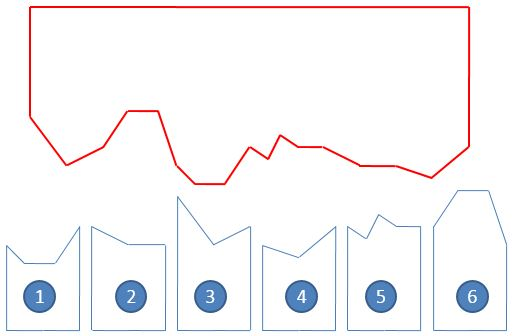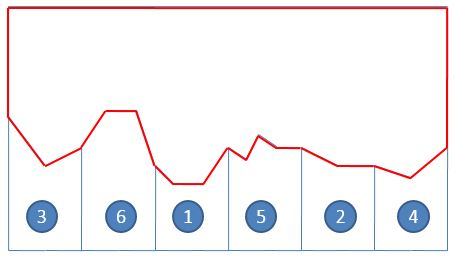### 输入格式：

``K h h ... h[K]``

### 输入样例：

``````17
95 70 80 97 97 68 58 58 80 72 88 81 81 68 68 60 80
6
4 68 58 58 80
3 81 68 68
3 95 70 80
3 68 60 80
5 80 72 88 81 81
4 80 97 97 68``````

### 输出样例：

``3 6 1 5 2 4``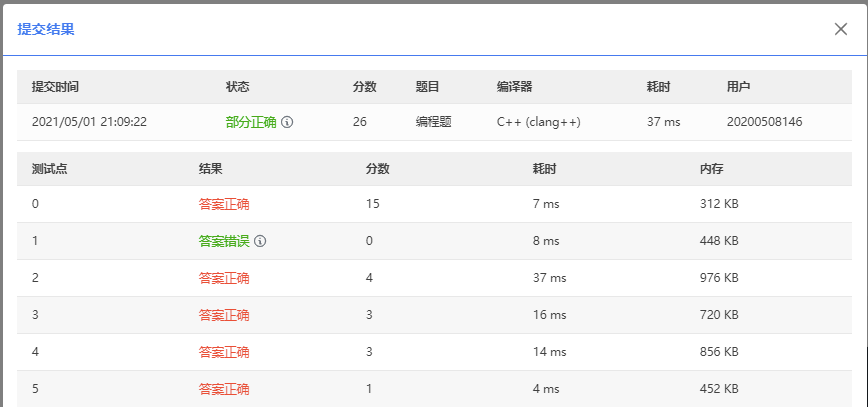### Python Excel操作模块XlsxWriter之写入日期worksheet.write_datetime()_xlsxwriter 日期_AuserBB的博客-程序员秘密

worksheet.write_datetime()write_datetime(row, col, datetime[, cell_format])向工作表单元格写入日期或时间。参数：row(int) - 单元格所在的行（索引从0开始计数）。col(int) - 单元格所在的列（索引从0开始计数）。datetime(datetime) - datetime.datetime， .date， .t...

### position和transform的用法_position transform_新参者-tangent的博客-程序员秘密

POSITIONCSS中我们想要改变一个元素的位置，我们可以采用的方法之一就是定位，这也是我们最先接触的方法。其主要用法就是给需要移动的元素的父元素或以上添加 position:relative; 再给自身添加 position:absolute; 然后通过改变方位（top，left，right，bottom）来实现元素的移动。TRANSFORMCSS3中提供了transform属性，其中的translateX和translateY值可以实现元素的横向与纵向移动。区别和选择经过测试。TRANSFO

### UE4 Hair Strands浅析_cosserat rod_kuangben2000的博客-程序员秘密

=============UE4 Hair Strands浅析https://zhuanlan.zhihu.com/p/128669105UE4.24 Hair Strands浅析UE4.24推出了次世代发丝（Hair Strands）系统Groom，其实现大量参考了寒霜在Siggraph2019 Advances in Real-Time Rendering in Games course上的演讲。 Strand-based Hair Rendering in Frostbite在写这篇文章

### HTML5新增加的功能_weixin_30955341的博客-程序员秘密

1、部分代码代替了以前的代码 例如：获取焦点 旧：document.getElementById("price");.focus; 新：&lt;input type="text" autofocus name="price" /&gt;2、使用HTML5之前，要表达一个文档的结构，只能通过&lt;div&gt;来实现；在HTMl5...

### BurpSuite的使用教程（下）_afei00123的博客-程序员秘密

5.Repeater(中继器/重放)功能模块 Repeater是用于手动操作和发送个别HTTP请求，并分析应用程序的响应一个简单的工具。可以发送一个内部请求从Burp任何地方到Repeater，修改请求并且发送。afei 可以从Proxy history、site map、Scanner等模块中右键菜单send to repeater发送到repeate...